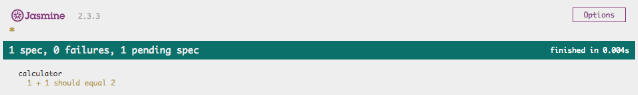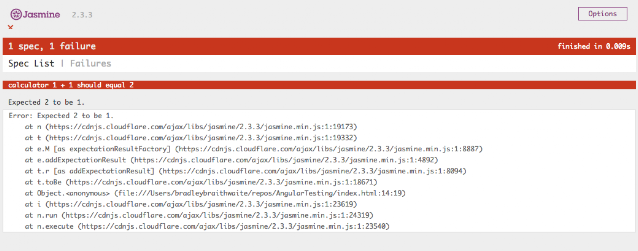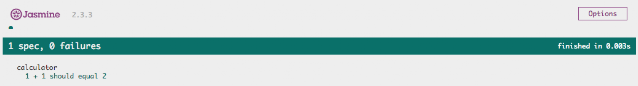### 前言

BBD和TBD两种风格会有些区别，但是使用`jasmine`确实可以用TBD的方式。`jasmine`会提供一种结构去组织你的测试以及一些函数来进行代码输出的断言。究竟是TBD还是BBD实际取决于开发者自己的如何去组织自己的测试，当然下文我们仅仅用TBD来进行讲解。

### 开始你的第一个测试

``````<html>
<script src="https://cdnjs.cloudflare.com/ajax/libs/jasmine/2.3.3/jasmine.min.js"></script>
<script type="text/javascript" src="https://cdnjs.cloudflare.com/ajax/libs/jasmine/2.3.3/jasmine-html.min.js"></script>
<script type="text/javascript" src="https://cdnjs.cloudflare.com/ajax/libs/jasmine/2.3.3/boot.min.js"></script>
<body>
</body>
<script type="text/javascript">

// Paste in the test code here.

</script>
</html>
````````````describe('calculator', function () {
it('1 + 1 should equal 2');
});
````````````it('1 + 1 should equal 2', function() {
expect(1 + 1).toBe(1);
});
````````````expect(true).toBe(true);
expect(false).not.toBe(true);
expect(1).toEqual(1);
expect('foo').toEqual('foo');
expect('foo').not.toEqual('bar');
``````

``````it('1 + 1 should equal 2', function() {
expect(1 + 1).toBe(2);
});
``````• 红色部分:失败的测试，有利于我们消除bug;
• 绿色部分：需要我们写足够的代码让我们的测试通过，透过前面的部分，我们可以了解这些bug;
• 黄色部分，代码重构过程我们可以避免重蹈覆辙，并且提升安全性。

### Demo

#### 写一个不通过的测试

``````describe('calculator', function () {

it('1 + 1 should equal 2', function () {
expect(calculator.sum(1, 1)).toBe(2);
});

});
``````

#### 2.使测试通过

``````var calculator = {
sum: function(x, y) {
return 2; // <-- note this is hardcoded
}
}

describe('calculator', function () {

it('1 + 1 should equal 2', function () {
expect(calculator.sum(1, 1)).toBe(2);
});

});
``````

#### 3.重构

``````var calculator = {
sum: function(x, y) {
return x + y;
}
}

describe('calculator', function () {

it('1 + 1 should equal 2', function () {
expect(calculator.sum(1, 1)).toBe(2);
});

});
``````

### 小结

You Can Speak "Hi" to Me in Those Ways